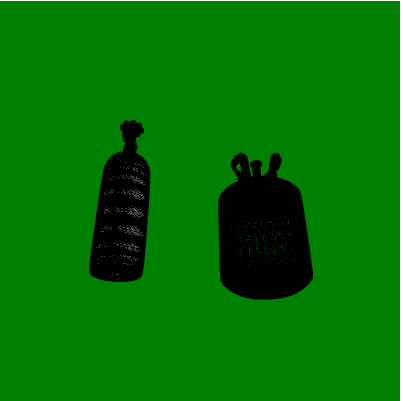Related Articles
p5.js preload() Function
• Last Updated : 22 Feb, 2021

The preload() function in p5.js is used to asynchronously load files before the setup() function. It runs exactly once in when the sketch is loaded.

Most users do not use the preload() function as the same task can be done in setup() function. However, it is nice to separate similar code in our program to improve its scalability and modularity. Generally preload() function is used to load things like, images, 3D models, fonts, etc. in the sketch. The “loading…” text is displayed during the loading of the resources.

Syntax:

```preload() {
// All loading calls here
}```

Parameters: This function does not accept any parameters.

Below example illustrates the preload() function in p5.js:

Example 1:

## Javascript

 `let gfg_img;``function` `preload() {`` ` `  ``// Loading the image``  ``gfg_img = loadImage(``'/gfg.png'``);``}`` ` `function` `setup() {``  ``createCanvas(400, 400);``}`` ` `function` `draw() {`` ` `  ``// Set background color to green``  ``background(``'green'``);`` ` `  ``// Show loaded image on screen at (100, 100)``  ``image(gfg_img, 100, 100);``}`

Output:Example 2:

## Javascript

 `let cylinder;``function` `preload() {`` ` `  ``// Loading a cylinder model``  ``cylinder = loadModel(``'/cylinder.stl'``, ``true``);``}`` ` `function` `setup() {``  ``createCanvas(400, 400, WEBGL);``  ``noLoop();``}`` ` `function` `draw() {`` ` `  ``// Set background color to green``  ``background(``'green'``);`` ` `  ``// Display and rotate the model``  ``rotateX(90);``  ``model(cylinder);``}`

Output:My Personal Notes arrow_drop_up